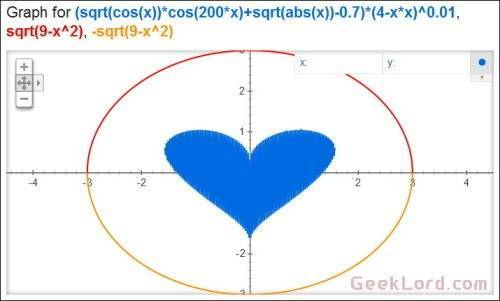`(sqrt(cos(x))*cos(200x)+sqrt(abs(x))-0.7)*(4-x*x)^0.01, sqrt(9-x^2), -sqrt(9-x^2) from -4.5 to 4.5`